5TH ANNIVERSARY AUCTION

Auction Details

Choose Your Layout >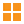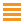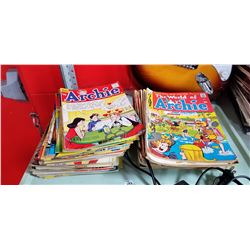252 - Shelf One
CDC Auctions
Shelf One
Bidding Has Concluded

Sold to f***r for (90.00 + 11.70) x 1 = 101.70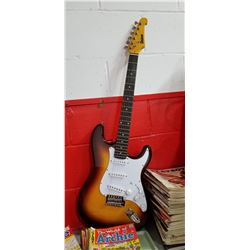253 - Shelf One
CDC Auctions
Shelf One
Bidding Has Concluded

Sold to f***r for (85.00 + 11.05) x 1 = 96.05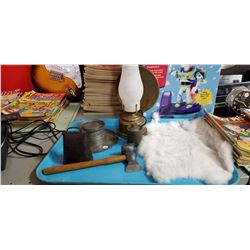254 - Shelf One
CDC Auctions
Shelf One
Bidding Has Concluded

Sold to f***r for (20.00 + 2.60) x 1 = 22.60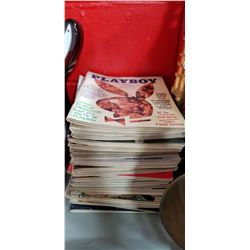255 - Shelf One
CDC Auctions
Shelf One
Bidding Has Concluded

Sold to s********F for (15.00 + 1.95) x 1 = 16.95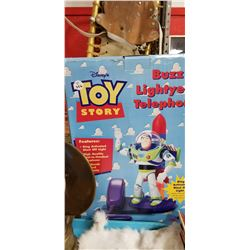256 - Shelf One
CDC Auctions
Shelf One
Bidding Has Concluded

Sold to f***r for (22.50 + 2.93) x 1 = 25.43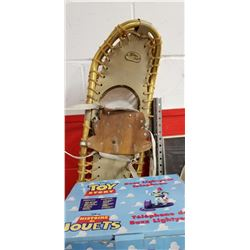257 - Shelf One
CDC Auctions
Shelf One
Bidding Has Concluded

Sold to f***r for (30.00 + 3.90) x 1 = 33.90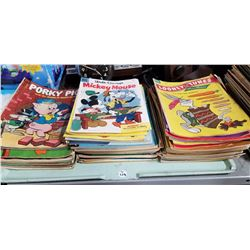258 - Shelf One
CDC Auctions
Shelf One
Bidding Has Concluded

Sold to f***r for (50.00 + 6.50) x 1 = 56.50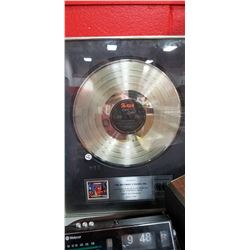260 - Shelf One
CDC Auctions
Shelf One
Bidding Has Concluded

Sold to f***r for (45.00 + 5.85) x 1 = 50.85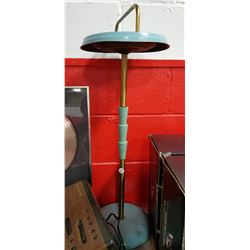261 - Shelf One
CDC Auctions
Shelf One
Bidding Has Concluded

Sold to f***r for (12.50 + 1.63) x 1 = 14.13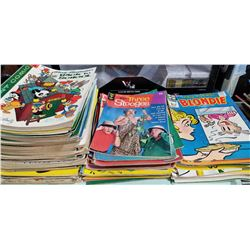262 - Shelf One
CDC Auctions
Shelf One
Bidding Has Concluded

Sold to f***r for (160.00 + 20.80) x 1 = 180.80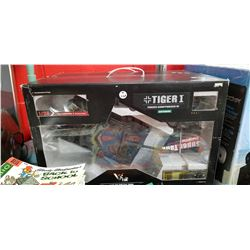263 - Shelf One
CDC Auctions
Shelf One
Bidding Has Concluded

Sold to f***r for (22.50 + 2.93) x 1 = 25.43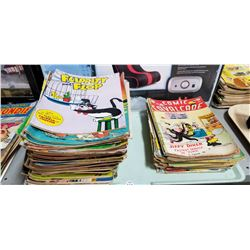264 - Shelf One
CDC Auctions
Shelf One
Bidding Has Concluded

Sold to f***r for (100.00 + 13.00) x 1 = 113.00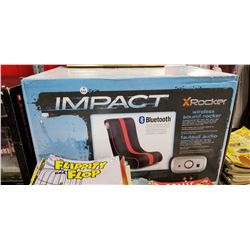265 - Shelf One
CDC Auctions
Shelf One
Bidding Has Concluded

Sold to f***r for (35.00 + 4.55) x 1 = 39.55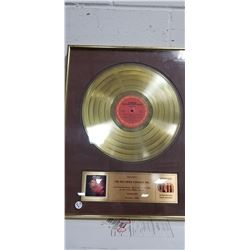266 - Shelf One
CDC Auctions
Shelf One
Bidding Has Concluded

Sold to f***r for (37.50 + 4.88) x 1 = 42.38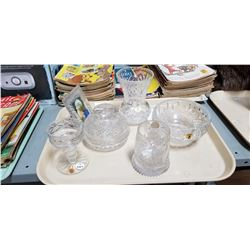267 - Shelf One
CDC Auctions
Shelf One
Bidding Has Concluded

Sold to f***r for (27.50 + 3.58) x 1 = 31.08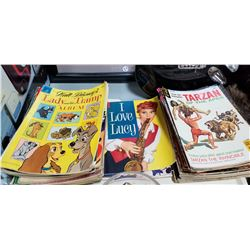268 - Shelf One
CDC Auctions
Shelf One
Bidding Has Concluded

Sold to f***r for (75.00 + 9.75) x 1 = 84.75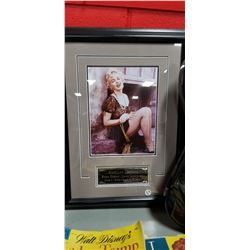269 - Shelf Two
CDC Auctions
Shelf Two
Bidding Has Concluded

Sold to f***r for (25.00 + 3.25) x 1 = 28.25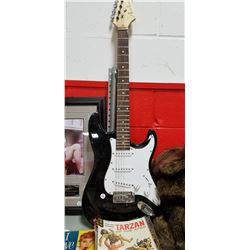270 - Shelf One
CDC Auctions
Shelf One
Bidding Has Concluded

Sold to f***r for (45.00 + 5.85) x 1 = 50.85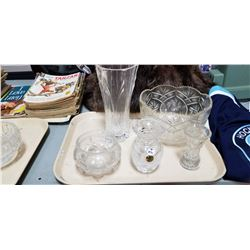271 - Shelf One
CDC Auctions
Shelf One
Bidding Has Concluded

Sold to f***r for (37.50 + 4.88) x 1 = 42.38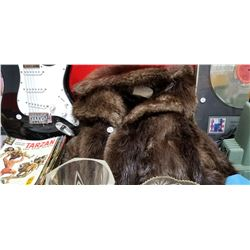272 - Shelf One
CDC Auctions
Shelf One
Bidding Has Concluded

Sold to f***r for (85.00 + 11.05) x 1 = 96.05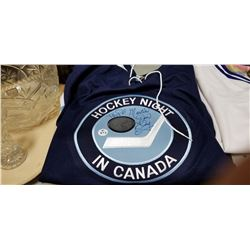273 - Shelf One
CDC Auctions
Shelf One
Bidding Has Concluded

Sold to f***r for (20.00 + 2.60) x 1 = 22.60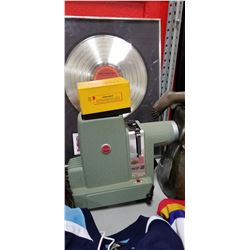274 - Shelf One
CDC Auctions
Shelf One
Bidding Has Concluded

Sold to f***r for (5.00 + 0.65) x 1 = 5.65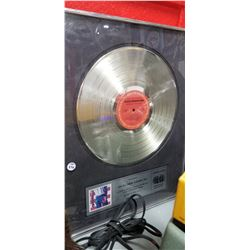275 - Shelf One
CDC Auctions
Shelf One
Bidding Has Concluded

Sold to f***r for (75.00 + 9.75) x 1 = 84.75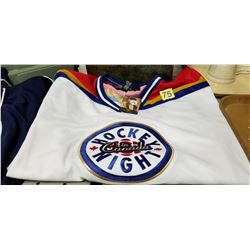276 - Shelf One
CDC Auctions
Shelf One
Bidding Has Concluded

Sold to s********F for (25.00 + 3.25) x 1 = 28.25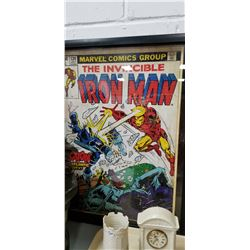278 - Shelf One
CDC Auctions
Shelf One
Bidding Has Concluded

Sold to f***r for (32.50 + 4.23) x 1 = 36.73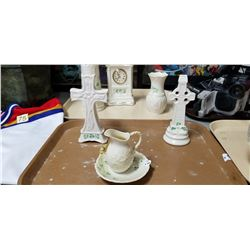279 - Shelf One
CDC Auctions
Shelf One
Bidding Has Concluded

Sold to f***r for (5.00 + 0.65) x 1 = 5.65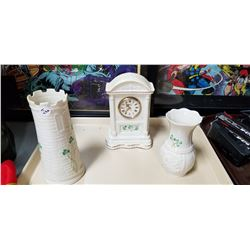280 - Shelf One
CDC Auctions
Shelf One
Bidding Has Concluded

Sold to f***r for (10.00 + 1.30) x 1 = 11.30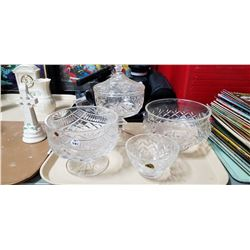281 - Shelf One
CDC Auctions
Shelf One
Bidding Has Concluded

Sold to f***r for (35.00 + 4.55) x 1 = 39.55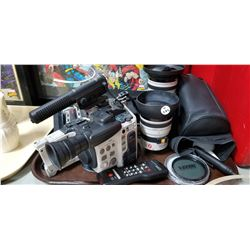282 - Shelf One
CDC Auctions
Shelf One
Bidding Has Concluded

Sold to f***r for (240.00 + 31.20) x 1 = 271.20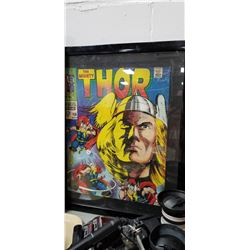283 - Shelf One
CDC Auctions
Shelf One
Bidding Has Concluded

Sold to f***r for (40.00 + 5.20) x 1 = 45.20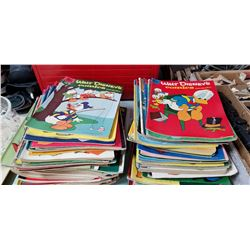284 - Shelf One
CDC Auctions
Shelf One
Bidding Has Concluded

Sold to f***r for (120.00 + 15.60) x 1 = 135.60285 - Shelf One
CDC Auctions
Shelf One
Bidding Has Concluded

Sold to f***r for (90.00 + 11.70) x 1 = 101.70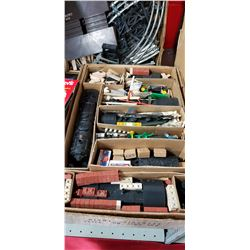286 - Shelf One
CDC Auctions
Shelf One
Bidding Has Concluded

Sold to f***r for (200.00 + 26.00) x 1 = 226.00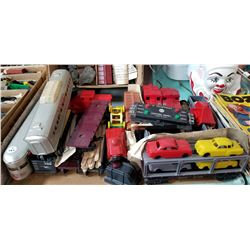287 - Shelf One
CDC Auctions
Shelf One
Bidding Has Concluded

Sold to f***r for (95.00 + 12.35) x 1 = 107.35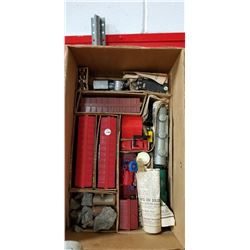288 - Shelf Two
CDC Auctions
Shelf Two
Bidding Has Concluded

Sold to f***r for (5.00 + 0.65) x 1 = 5.65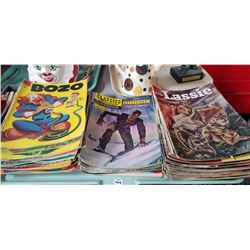289 - Shelf One
CDC Auctions
Shelf One
Bidding Has Concluded

Sold to f***r for (65.00 + 8.45) x 1 = 73.45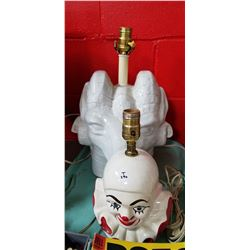290 - Shelf One
CDC Auctions
Shelf One
Bidding Has Concluded

Sold to f***r for (20.00 + 2.60) x 1 = 22.60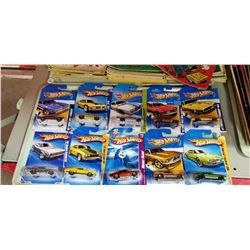292 - Shelf One
CDC Auctions
Shelf One
Bidding Has Concluded

Sold to p*******1 for (10.00 + 1.30) x 1 = 11.30293 - Shelf One
CDC Auctions
Shelf One
Bidding Has Concluded

Sold to f***r for (140.00 + 18.20) x 1 = 158.20294 - Shelf One
CDC Auctions
Shelf One
Bidding Has Concluded

Sold to d******G for (110.00 + 14.30) x 1 = 124.30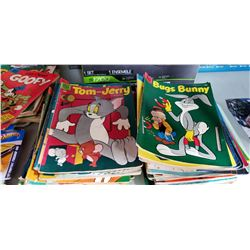295 - Shelf One
CDC Auctions
Shelf One
Bidding Has Concluded

Sold to f***r for (55.00 + 7.15) x 1 = 62.15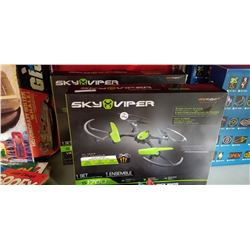296 - Shelf One
CDC Auctions
Shelf One
Bidding Has Concluded

Sold to f***r for (50.00 + 6.50) x 1 = 56.50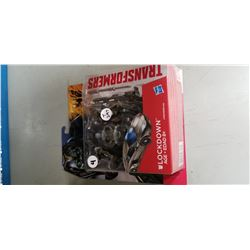297 - Shelf One
CDC Auctions
Shelf One
Bidding Has Concluded

Sold to s********F for (20.00 + 2.60) x 1 = 22.60